# AI悦创·编程一对一教学（Python 一级）等级考试试卷

AI悦创·推出辅导班啦，包括「Python 语言辅导班、C++辅导班、算法/数据结构辅导班、少儿编程(Scratch 等）、pygame 游戏开发」，全部都是一对一教学：一对一辅导 + 一对一答疑 + 布置作业 + 项目实践等。QQ、微信在线，随时响应！V：Jiabcdefh

## 一、单选题(共25题，每题2分，共50分)

1. 以下哪种输入结果不可能得到以下反馈： 重要的事情说三遍：安全第一！安全第一！安全第一！（ ）

​ A. print(“重要事情说三遍：”+“安全第一！”*3)

​ B. print(“重要事情说三遍：”+“安全第一！“+”安全第一！”*2)

​ C. print(“重要事情说三遍：”+“安全第一！”+“安全第一！”+“安全第一！”)

​ D. print(“重要事情说三遍：”+“安全第一！”/3)

1. 运行下列程序后，绘制出以下哪个图形？（ ）
import turtle
turtle.pensize(3)
turtle.forward(150)
turtle.circle(50,180)
turtle.forward(180)
turtle.circle(48,180)
turtle.forward(150)
turtle.circle(45,180)
turtle.forward(120)
turtle.done()


A.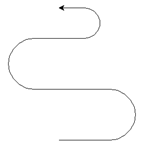B.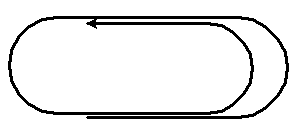C.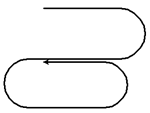D.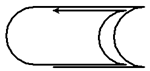1. 执行 print(1 + 2 * 2+ 6 / 3) 的结果为?（ ）

​ A. 4

​ B. 7

​ C. 4.0

​ D. 7.0

1. 已知变量 x=2，语句 print(“x=”,x) 的作用是？（ ）

​ A. 在屏幕上输出 x=x

​ B. 在屏幕上输出 2=2

​ C. 在屏幕上输出 x=2

​ D. 在屏幕上输出 “x=” 2

1. 执行下面程序后，画布上会出现几只海龟？（ ）
import turtle

t1=turtle.Turtle('turtle')
t2=turtle.Turtle('turtle')
t3=turtle.Turtle('turtle')
t4=turtle.Turtle('turtle')

t1.forward(50)
t2.forward(100)
t3.forward(150)
t4.forward(200)


A. 0

B. 1

C. 4

D. 5

1. print(24%5), 运算结果是？（ ）

​ A. 1

​ B. 2

​ C. 3

​ D. 4

1. 下面哪个指令不可以让海龟回到坐标（0，0）点？（ ）

​ A. turtle.goto(0,0)

​ B. turtle.home()

​ C. turtle.setposition(0,0)

​ D. turtle.set(0,0)

1. 以下程序输出的结果是？（ ）
a=30
b=5
print(a/b)


​ A. 6

​ B. 30/5

​ C. 6.00

​ D. 6.0

1. print(46//8)的结果是？（ ）

​ A. 5

​ B. 6

​ C. 5.7

​ D. 5.75

1. Python启动后显示的提示符是？（ ）

​ A. c:>

​ B. >>>

​ C. —

​ D. %%%

1. 下列代码不能画出直径为 10 的点的是?（ ）

A.

turtle.pensize(10)
turtle.pendown()


B.

turtle.dot(10)


C.

turtle.begin_fill()
turtle.circle(5)
turtle.end_fill()


D.

turtle.begin_fill()
turtle.circle(10)
turtle.end_fill()

1. 已知变量 a=5，b=6，执行语句 a*=a+b 后，变量 a 的值为：（ ）

​ A. 11

​ B. 30

​ C. 31

​ D. 55

1. 如果 a=23，b=10，那么 print(a%b) 的结果是？（ ）

​ A. 2

​ B. 3

​ C. 23

​ D. 2.3

14. 下列表达式的值为 True 的是？（ 	）


​ A. ‘a’>‘b’

​ B. 2>3

​ C. ‘A’>‘a’

​ D. ‘3’>‘2’

1. 已知 x=5，y=6，则表达式 not(x!=y) 的值为：（ ）

​ A. True

​ B. False

​ C. 5

​ D. 6

1. 执行下面程序后，以下哪个图形是正确的？（ ）
import turtle
turtle.shape('square')
turtle.home()
turtle.dot()
turtle.stamp()
turtle.forward(100)
turtle.stamp()
turtle.forward(100)
turtle.left(90)
turtle.stamp()
turtle.forward(100)
turtle.left(90)
turtle.stamp()
turtle.forward(100)


​ A.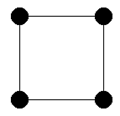​ B.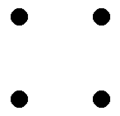​ C.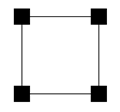​ D.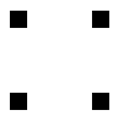1. 输出如下古诗，请问哪句是正确的？（ ）

A.

print(
'闻道梅花坼晓风，雪堆遍满四山中。
何方可化身千亿，一树梅花一放翁。')


B.

print('闻道梅花坼晓风，雪堆遍满四山中。'
'何方可化身千亿，一树梅花一放翁。')


C.

print('''闻道梅花坼晓风，雪堆遍满四山中。



D.

print("闻道梅花坼晓风，雪堆遍满四山中。"\n
"何方可化身千亿，一树梅花一放翁。")

1. 执行以下两段代码
a=123
print(a%100%10)


​ A. 1

​ B. 2

​ C. 3

​ D. 1.23

1. 下面哪个程序，最有可能得到下面这个图形？（ ）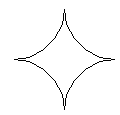A.

turtle.circle(50,90)
turtle.circle(-50,-90)
turtle.circle(50,90)
turtle.circle(-50,-90)

B.

turtle.circle(50,90)
turtle.circle(-50,-90)
turtle.circle(-50,-90)
turtle.circle(50,90)

C.

turtle.circle(50,90)
turtle.circle(50,90)
turtle.circle(-50,-90)
turtle.circle(-50,-90)

D.

turtle.circle(-50,-90)
turtle.circle(50,90)
turtle.circle(50,90)
turtle.circle(-50,-90)

1. 下面描述中，不符合 Python 语言特点的是：（ ）

​ A. Python 是一门面向对象的编程语言

​ B. Python 程序通过编译后执行

​ C. Python 支持函数编程

​ D. Python 支持多个操作系统

1. 下列哪个不是 Python 的保留字？（ ）

​ A. if

​ B. or

​ C. do

​ D. for

1. 执行下列语句后的显示结果是什么？（ ）
b = 2 * a / 4
a = 1
print(a,b)


​ A. 1 , 0.5

​ B. 1 , 0

​ C. 报错

​ D. 0 , 1

a=5
print(‘a+4')


​ A. 9

​ B. ‘a+4’

​ C. 无结果，出错

​ D. a+4

1. 以下选项中，Python 语言中代码注释使用的符号是？（ ）

​ A. /… …/

​ B. ！

​ C. #

​ D. //

1. 在 turtle 库中的指令，执行以下代码指令后，走出的一个正方形形状，此时海龟的面朝方向应该是往哪里？（ ）

import turtle

turtle.goto(0,0)

turtle.goto(0,100)

turtle.goto(100,100)

turtle.goto(100,0)

turtle.goto(0,0)

​ A. 水平向左

​ B. 水平向右

​ C. 垂直向上

​ D. 垂直向下

## 二、判断题(共10题，每题2分，共20分)

1. 语句：

print( “a”, end=" " )

print(“b”, end=" " )

1. print() 函数不可以在屏幕上打印出空行。

1. Turtle 库中，使用 circle(20) 命令，指的是画出以画布正中央为圆心，半径为 20 的圆形。

1. 在 IDLE 编辑器中，Python 代码的字体和字号可以根据需要自行设置，方便大家的使用。

1. Abc、aBc、abC 是三个不同的变量。

1. one,two,three=‘1’,3,5; print(one+two+three)运行结果是9。

1. a*=b，就是 a=b*b。

1. Python 除了用自带的 IDLE 进行编程外还可以用其他编程环境进行程序编写，比如 JupyterNotebook。

1. turtle.circle(50,steps=5)命令可以画一个五角星。

1. is 和 input 都是关键字，不能随意使用。

## 三、编程题(共3题，共30分)

1. 画出下面示意图形，要求如下：

（1）画出如下的图形，注：直线部分是由两个步长为 200 的线段垂直相交组成，

（2）圆的直径为200；

（3）图形的中心位置为画布中心；

（4）画笔宽度为2，颜色为红色。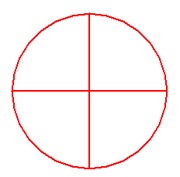1. 已知一头奶牛每天可以产 20 千克牛奶。N(N为变量)头奶牛 7 天可以产多少千克的牛奶？

要求：

（1）程序开始运行后，会有提示字符串：“请输入奶牛的头数：”，完成奶牛头数的输入；

（2）（可以直接赋值提示字符串到程序中，提示字符串包括冒号，但不包括双引号）

（3）程序会根据输入的奶牛头数计算出总共产出的牛奶的重量，并将结果进行修饰然后

（4）输出。示例：如果输入奶牛的头数为10，则输出“10 头奶牛7天可以产 1400 千克的牛奶”

2. Python一级 面试题

3. 本题不需要作答；

4. 请考生进入面试间等待叫号进行面试，由主考官提问问题，考生回答。

AI悦创·推出辅导班啦，包括「Python 语言辅导班、C++辅导班、算法/数据结构辅导班、少儿编程(Scratch 等）、pygame 游戏开发」，全部都是一对一教学：一对一辅导 + 一对一答疑 + 布置作业 + 项目实践等。QQ、微信在线，随时响应！V：Jiabcdefh12-021569
08-072409
03-215040
05-09908
03-161134
06-155965
10-306349
12-101万+
12-012130
12-31
08-043022
04-23791
04-19120
05-18261
08-291万+

### “相关推荐”对你有帮助么？

•非常没帮助
•没帮助
•一般
•有帮助
•非常有帮助AI悦创

¥2 ¥4 ¥6 ¥10 ¥20余额支付 (余额：-- )扫码支付获取中扫码支付点击重新获取扫码支付1.余额是钱包充值的虚拟货币，按照1:1的比例进行支付金额的抵扣。
2.余额无法直接购买下载，可以购买VIP、C币套餐、付费专栏及课程。余额充值